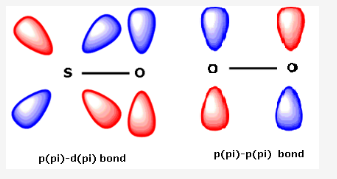# What are p pi p pi bonds and p pi d pi bonds?

What are p pi p pi bonds and p pi d pi bonds?

If there is bonding between two atoms where one atom is having one vacant orbital and another is having one lone pair of electrons, then if this electron pair is donated to that respective vacant orbital then the bonding is called p(pi) - p(pi) or p(pi) - d(pi) depending on the orbital to which the electron pair is donated and from which the electron pair is donated.

For ex: Nitrogen can not form p(pi) - d(pi) bond because of absence of d-orbitals in its valence shell. But Phosphorus, Arsenic etc can form p(pi) - d(pi) bonds. If we consider the bonding in case of SO2, S atom undergoes sp2 hybridisation. Here, the multiple bonding is due to p(pi) - p(pi) or p(pi) - d(pi) bonding. The vacant 3d-orbitals of S overlap with the filled 2p orbitals of O.